Many Proofs of Pythagorean Theorem

### Many Proofs of Pythagorean Theorem

List of animations posted on this page.(Click the text to watch animation.)See Euclid's classical solution in animation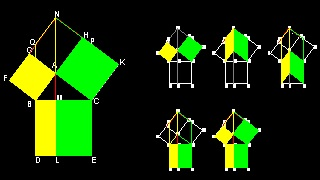See Rectangle variation by Lecchio (1753) in animation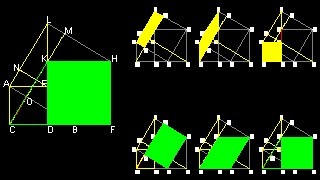See Yanney's proof (1903) in animation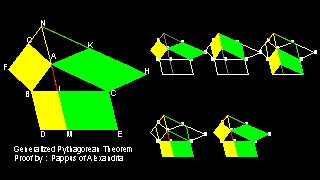See Generalization to parallelogram by Pappus of Alexandria in animation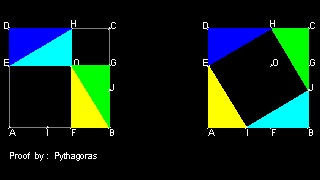See Graphical proof by Pythagoras in animation

Go to Proof by Pythagoras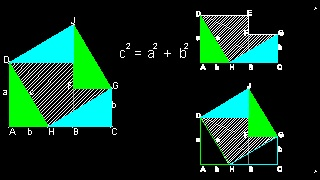See Perigal's dissection (1873) in animation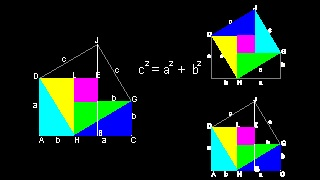See variation of Perigals' dissection proof in animation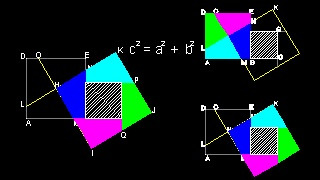See Dudeney's construction of 1917 in animation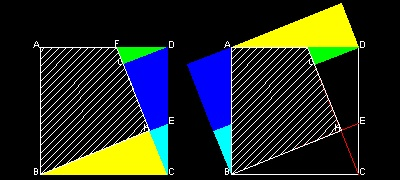See Hisashi Abe's origami solution-1 in animation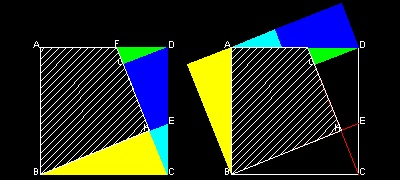See Hisashi Abe's origami solution-2 in animation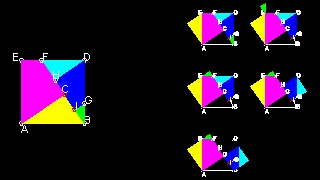See Mario Pacek's dynamic solution in animation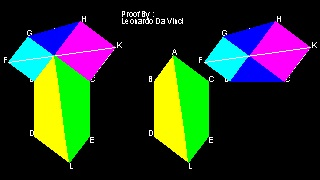See Leonardo Da Vinci's proof in animation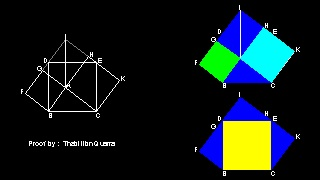See Thâbit ibn Quarra's proof in animation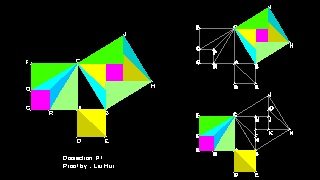See Liu Hui's dissection in animation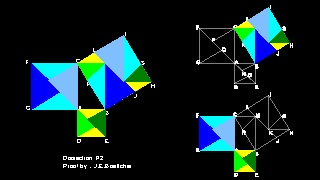See J.E.Böttcher's proof in animation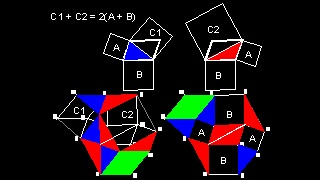See Generalization to parallelogram in animation

Probably the most famous theorem of all Geometry studies is the "Pythagorean Theorem". The name came from the famous Greek mathematician Pythagoras of Samos(circa 569-475 BC)
who was a spiritual leader of the group studying mathematics.

In Book I of Euclid's Element, this theorem is stated as Proposition 47.

"In right angled triangles the square on the side subtending the right angle

is equal to the squares on the sides containing the right angle."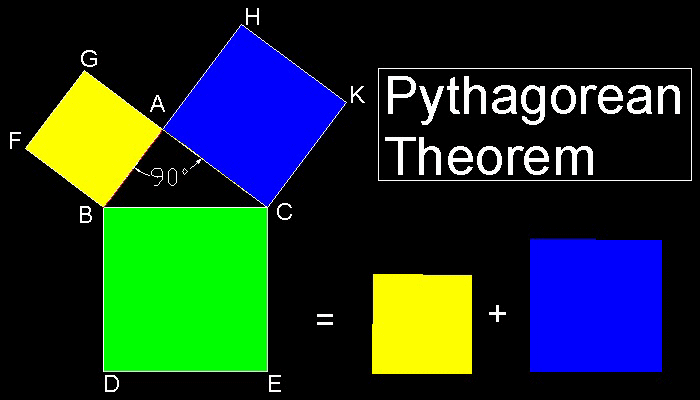***************************** Euclid_prop_47.dwg *****************************
```There are far more proofs offered of Pythagoras' theorem than of any other proposition in mathematics.
"The Pythagorean Proposition" published by Elisha Scott Loomis in 1940 contains 367 proofs !.
```

#### Proof by Euclid in "Element"***************************** Pythagorean_theorem_01.dwg *****************************

You can see the process in animation.

```This is one of the most elegant of many proofs. The center of the argumnet is that the
areas of triangles ACK, BCK , ACE and MCE are exactly the same. Therefore the areas of ACKH and MCEL
,which are twice that of triangle ACK & MCE respectively, are the same.
Similar argument is true for yellow colored parts, i.e.,ABFG & BMLD.
```

To create this drawing and animation:
Then from command line, type Euclid_47_1

```Following two proofs use rectangle and square instead of triangle.
```

#### Variation of Euclid's Proof #1

Ref. 1 (Elisha Scott Loomis) lists this proof as Geometric Proof #42, published by Lecchio in 1753.***************************** Pythagorean_theorem_05.dwg *****************************

You can see the process in animation.

To create this drawing and animation:
Then from command line, type Euclid_47_3

#### Variation of Euclid's Proof #2

B.F.Yanney published this proof in 1903 (Ref. 6) (p.283).
This is very similar to #8 .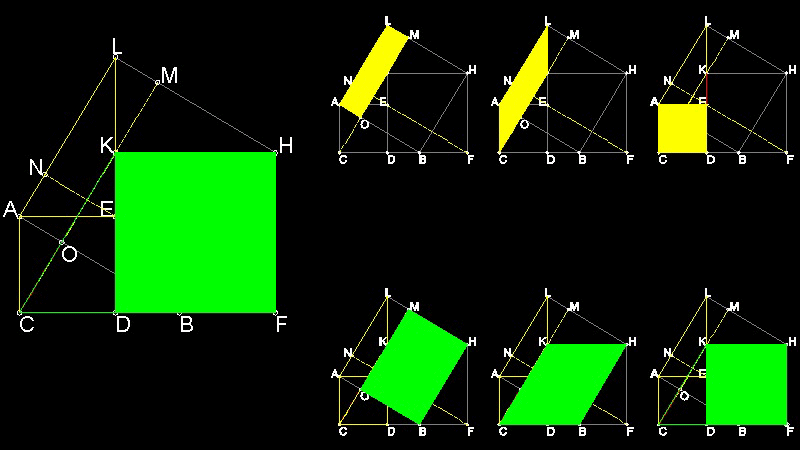***************************** Pythagorean_theorem_11.dwg *****************************
```Areas of LMOA = LKCA = ACED  and similarly, areas of HBOM = HBCK = HFDK
Hence ABHL = LMOA + HBOM = ACDE + HFDK
```

You can see the process in animation.

To create this drawing and animation:
Then from command line, type Euclid_47_9

#### Generalization by Pappus of Alexandria (Ref. 3)

In the figure NA = LM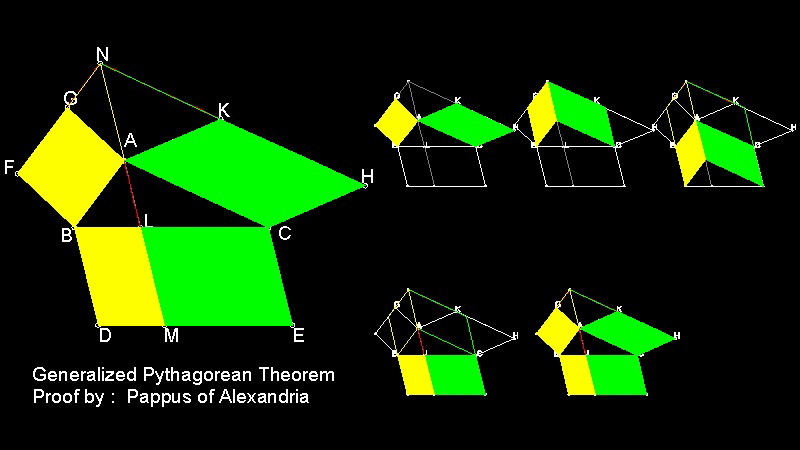***************************** Pythagorean_theorem_06.dwg *****************************

You can see the process in animation.

To create this drawing and animation:
Then from command line, type Euclid_47_4

```Pythagoras also offered a very simple proof.
```

#### Proof by Pythagoras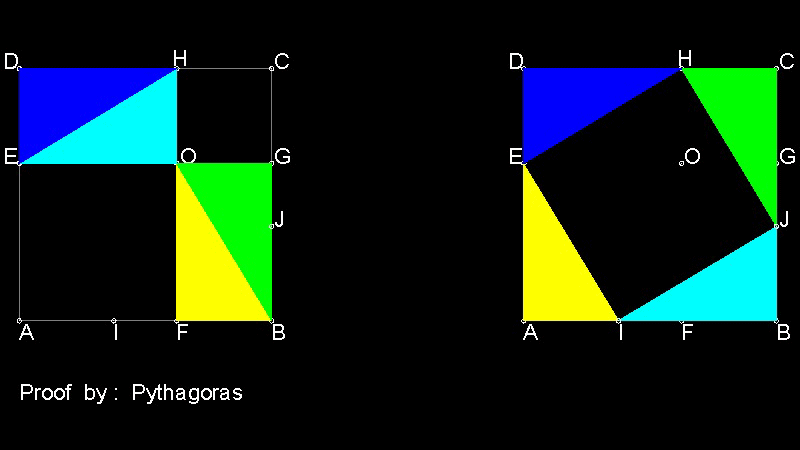***************************** Pythagorean_theorem_09.dwg *****************************

You can see the process in animation.

To create this drawing and animation:
Then from command line, type Euclid_47_7

#### General Concept of Dissection

There are many clever proofs using dissections. The general concept for using dissection can be explained as follows: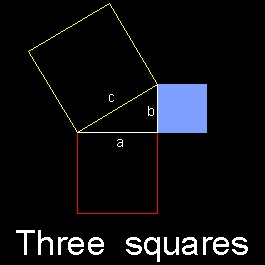****** tesselation_1.dwg ****** ****** tesselation_2.dwg ****** ****** tesselation_3.dwg ******

(1)In this example, the size of three squares a,b & c are given.(tesselation_1.dwg)
(2)Create a gapless pattern made up of two squares (a & b).(tesselation_2.dwg)
(3)Create a mesh like pattern using "c" on some transparent paper.(tesselation_3.dwg)
Then move the "mesh-C" over the pattern a-b so that the corner of mesh-C matches some characteristic points like center or edge of squares.

example follows:***************************** Pythagorean_theorem_12.dwg *****************************

To create this drawing :
Then from command line, type dissection

```Any combination of triangles and quadrilaterals cut out by the yellow square will make up
two small squares. So this method will create infinite number of dissection.
There are two interesting cases,i.e. center of squares and edge of squares.
```

#### Corner of smaller square-b is chosenTo create this drawing :
Then from command line, type dissection
Then match the corner point to the corner of smaller square.

This is Perigal's dissection of 1873.
*********** dissection_case_2.dwg ***********

#### Perigal's dissection of 1873***************************** Pythagorean_theorem_02.dwg *****************************

You can see the process in animation.

To create this drawing and animation:
Then from command line, type Euclid_47_2b

```The cross-hatched area in Perigal's dissection can be further divided into one square and
2 triangles. This leads us to the next variation.
```

#### Variation of Perigal's dissection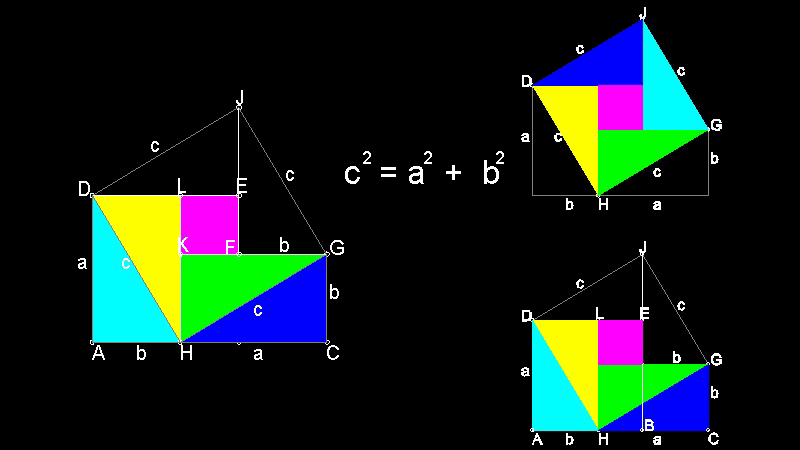***************************** Pythagorean_theorem_03.dwg *****************************

You can see the process in animation.

To create this drawing and animation:
Then from command line, type Euclid_47_2c

#### Center of larger square-a is chosen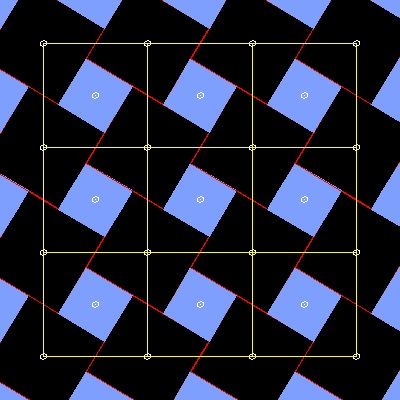To create this drawing :
Then from command line, type dissection
Then select the center of the larger square(b x b).

This is Henry Dudeney's construction of 1917.
************ dissection_case_3.dwg ************

#### Henry Dudeney's construction of 1917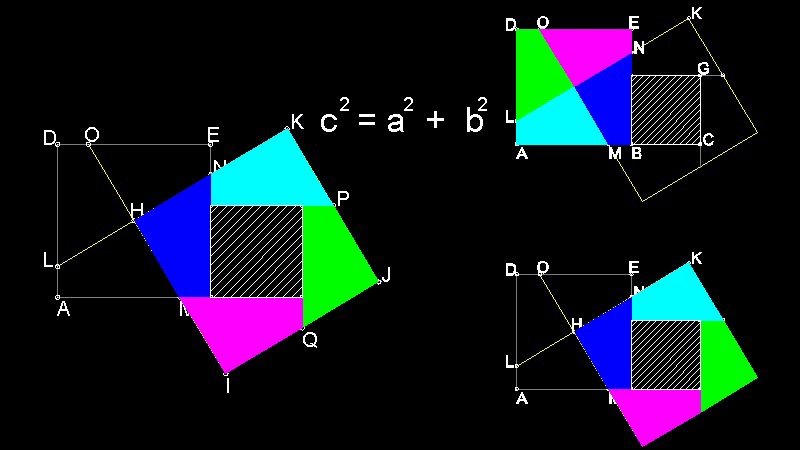***************************** Pythagorean_theorem_04.dwg *****************************

You can see the process in animation.

To create this drawing and animation:
Then from command line, type Euclid_47_2d

#### Corner of smaller square-b is chosen-2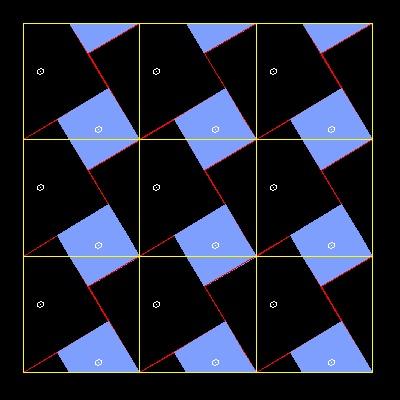When #5-a case is turned upside down,
and move the yellow frame to a different corner
point of smaller square,
then another interesting dissection is found.

#### Origami solution by Hisashi Abe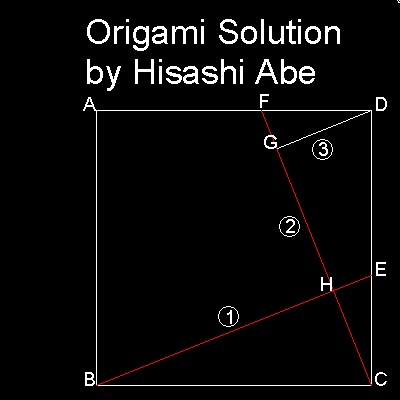Origami process:Prepare a square piece of paper.

```1. fold along line BE.
2. fold line CF by locating point E on line BE.
This makes CF perpendicular to BE.
3. fold line GD by locating F on line CF.
GD is now perpendicular to CF.
Note here that BCH and CDG are identical,
and GD = CH.
```
Here, this process is done by computer.

To create this drawing :
Then from command line, type Abe_origami
************* Abe_origami_0.dwg *************

```Cut out two trianlges BCH and DGC.
Move BCH upward so that line BC matches line AD.
Move DGC left so that line DC matches line AB.
```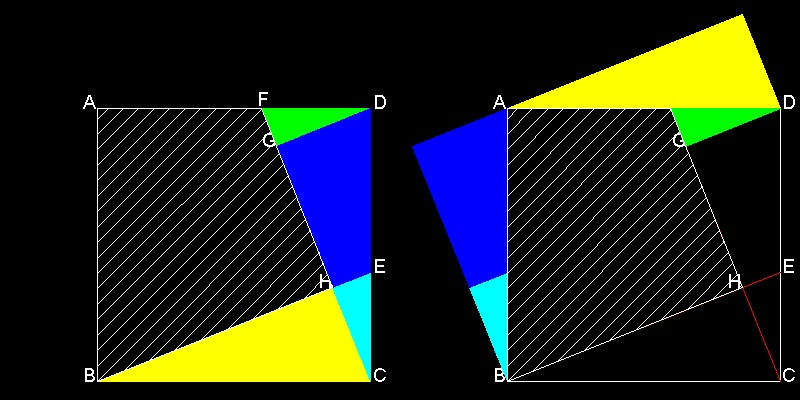***************************** Abe_origami_a.dwg *****************************

You can see the process in animation.

To create this drawing and animation:
Then from command line, type Abe_origami_a

```Since BCH = CDG, essentially the same result can be achieved by rotating these trianlges.
i.e. rotating BCH around point B, and CDG around point D.
```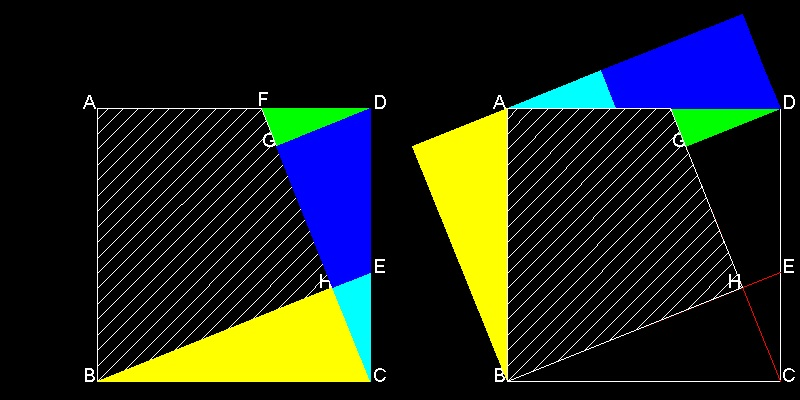***************************** Abe_origami_b.dwg *****************************

You can see the process in animation.

To create this drawing and animation:
Then from command line, type Abe_origami_b

```But this picture does not look neat.
The reason is the gap between two small triangles,green and cyan.
The picture would look nice and clean if cyan colored triangle
and green colored triangle meet at point F.
The following solution by Mario Pacek does just that.
```

#### Dynamic proof by Mario Pacek

I found this interesting proof in Pythagorean Theorem by Alexander Bogomolny.
Dr.Bogomolny says that this proof was done by Mario Pacek (aka Pakoslaw Gwizdalski).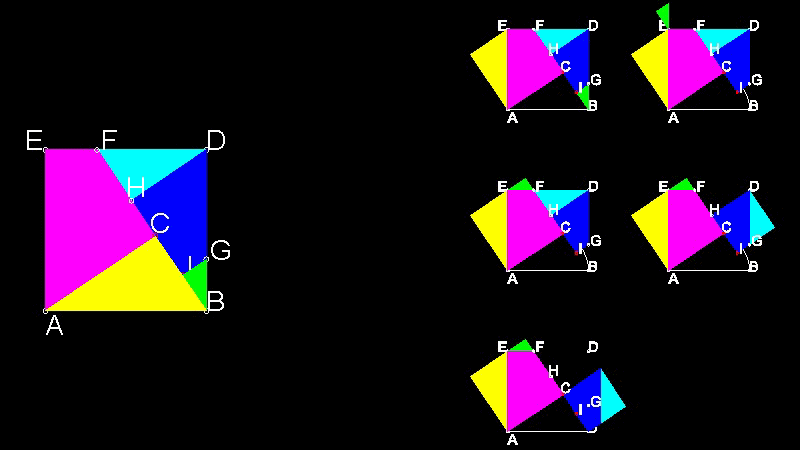***************************** Pythagorean_theorem_10.dwg *****************************

You can see the process in animation.

To create this drawing and animation:
Then from command line, type Euclid_47_8

### More of innovative proofs

#### Proof by Leonardo Da Vinci

This is a very famous proof by Leonardo Da Vinci(1452-1519).(Ref.3)(p. 31)***************************** Pythagorean_theorem_07.dwg *****************************

You can see the process in animation.

To create this drawing and animation:
Then from command line, type Euclid_47_5

#### Proof by Thâbit ibn Quarra(828-901)

This proof is by Thâbit ibn Qurra(828-901)(Ref.6)(p 282-283). Ref.6 gives a detailed discussion of his works.***************************** Pythagorean_theorem_08.dwg *****************************

You can see the process in animation.

To create this drawing and animation:
Then from command line, type Euclid_47_6

#### Proof by Liu Hui(3rd century AD) using Dissection

His proof was only in description ,so researchers tried to reconstruct
his idea. The following is one of them.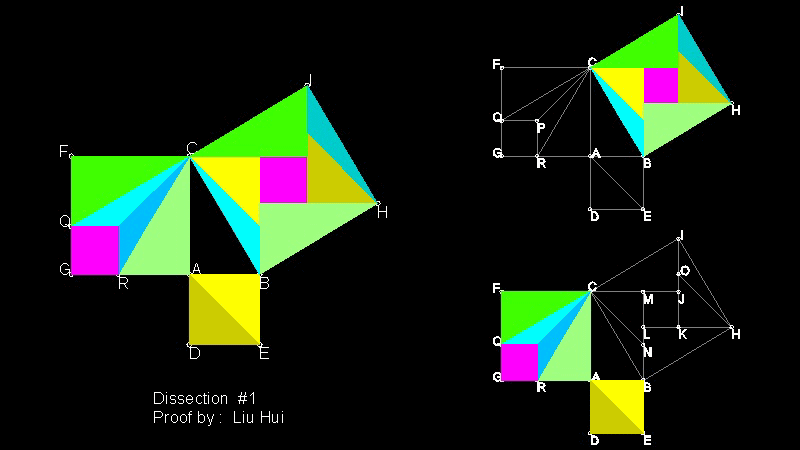***************************** Pythagorean_theorem_13.dwg *****************************

You can see the process in animation.

To create this drawing and animation:
Then from command line, type dissect_1

#### Proof by J.E.Böttcher using Dissection

Ref.1 lists the following dissection proof as Geometric Proofs #19 (p. 112) and
gives credit to Johannes Eduard Böttcher (1847 - 1919).***************************** Pythagorean_theorem_14.dwg *****************************

You can see the process in animation.

To create this drawing and animation:
Then from command line, type dissect_2

#### Generalized Pythagorean Theorem for a Parallelogram

This proof is published by David S. Wise (Ref. 9), and it was reprinted in Ref. 5 (p.9).
On Sept 14, 2011, the author ,David S. Wise, kindly notified me by e-mail as follows:

"I later found out that it was already known as Apollonius's parallelogram theorem."

When the parallelogram is a rectangle, this is Pythagorean theorem.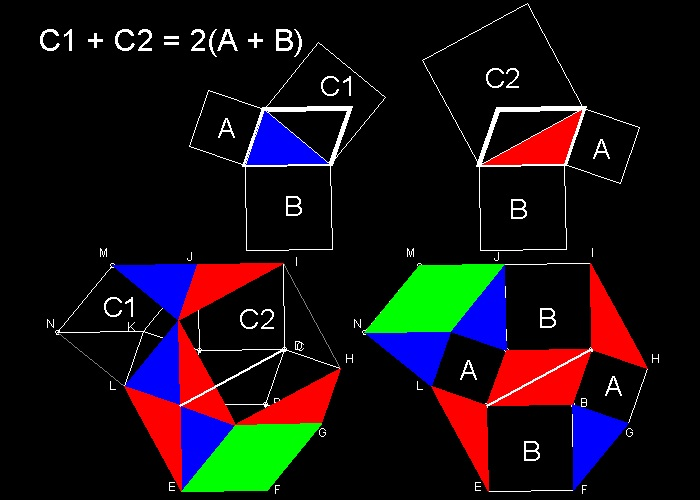***************************** Pythagorean_theorem_15.dwg *****************************

You can see the process in animation.

To create this drawing and animation:
Then from command line, type gen_Pythagoras

#### De Gua's Theorem: Generalization of the Pythagorean theorem to three dimensions

De Gua's theorem is a generalization of the Pythagorean theorem to three dimensions and named for Jean Paul de Gua de Malves.(1712-1785)
This theorem was presented to the Paris Academy of Sciences in 1783.
If a tetrahedron has a right angle corner (a corner like a cube), then the square of the area of the face opposite the right angle corner is the sum of the squares of the areas of the other three faces.

```  Then De Gua's theorem states
(Area of ABC)2= (area of OAB)2 + (area of OBC)2 + (area of OCA)2
Let OA = a, OB = b and OC = c.
Then this theorem can be written as follows:
(Area of ABC)2= (1/4){(ab)2 + (bc)2 + (ca)2}
One easy way to prove this is the use of Heron's formula for the area
of a triangle.
```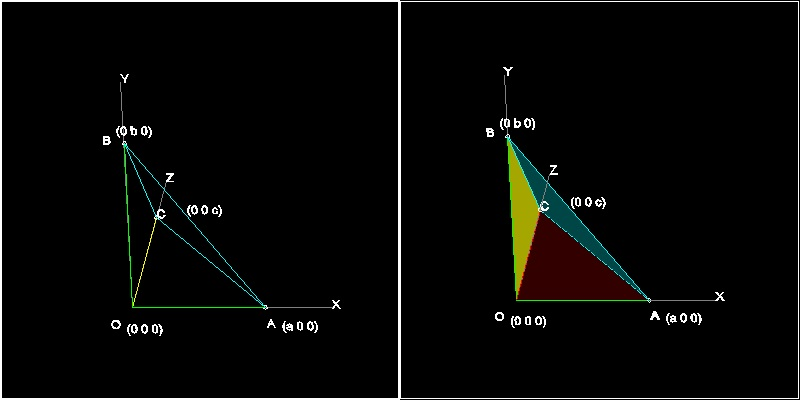*********************************** DeGua_model.dwg ***********************************

To create this drawing :
Then from command line, type DeGua

#### Spherical Pythagorean Theorem

For a right triangle ABC on a sphere of radius R with a right angle at vertex C
and sides of length a,b,and c, then

```	cos(c/R) = cos(a/R) * cos(b/R) .
```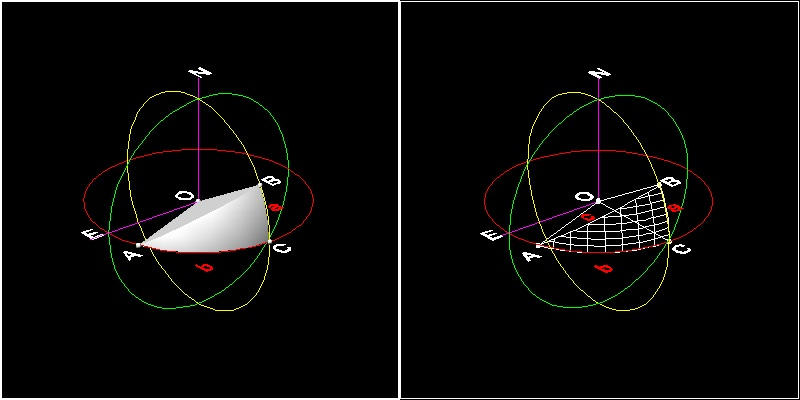******************************* spherical_Pythagorean.dwg ********************************

red great circle represents equator, yellow one represents longitude line of zero and 180, and
green color represents longitude=90 degrees line.
Point A : 100 deg East and on the equator
Point B : 180 deg East and 30 deg North
Point C : 180 deg East and on the equator
Radius of the Earth is chosen to be 6373 km(=3956 mile).
To create this drawing :
Then from command line, type sphere_pythagorean

```Spherical Pythagoreantheorem states:
cos(c/R) = cos(a/R) * cos(b/R)              (1)
Maclaurin series for cos(x) is :
cos(x) = 1 - x2/2! + x4/4! - x6/6! + ...
Using this formula in (1) gives us:

1 - (c/R)2/2 + (c/R)4/24 -...=
{1 - (a/R)2/2 + (a/R)4/24 -...}{1 - (b/R)2/2 + (b/R)4/24 -...}
=1 - (a/R)2/2 - (b/R)2/2 + (ab)2/(4 R4) +...

Or
c2 + (terms of higher powers of c)/R2
= a2 + b2 + (terms of higher powers of a & b)/R2
When R is large relative to a,b and c, then terms divided by R2 becomes negligibly small,
and we have a classical Pythagorean theorem ,i.e.
c2 = a2 + b2
```
##### Number check on this model
From the drawing shown above , it is possible to obtain arc length a,b and c.
Keep exploding the 3D objects until it is not possible to explode anymore.
Then use LIST command to get arc length.
This is the result.
```	a = 3333.7534		b = 7778.7579		c = 8086.6416	and R = 6367
Use these values to compute cos(a/R),etc.
Just run the command  check_theorem, and the results are
cos(a/R) = 0.866025
cos(b/R) = 0.34202
So cos(a/R)*cos(b/R) = 0.296198
This is very, very close the value of cos(c/R)
cos(c/R) = 0.296198

Finally*
cos(c/R)/{cos(a/R)*cos(b/R)} = 0.99999996
And for reference,
c2/{a2 + b2} = 0.91302773

*Note:This results are based on the number extracted from the graphical objects.
If all the length of a,b and c are computed using the trigonometry,
numerical precision is much higher (up to at least 12-th decimal place).

```

#### On Internet

1. Pythagoras' Theorem, by Bill Casselman, The University of British Columbia.
2. Many more interesting proofs by Alexander Bogomolny

3. A proof of the Pythagorean Theorem by Liu Hui (third century AD)
reference for dissection Proof #9

Go to   Fun_Math Content Table

All questions/complaints/suggestions should be sent to takaya.iwamoto@comcast.net

Last Updated July 9-th, 2006